# Properties of REAL Numbers Opposites Two real numbers

• Slides: 22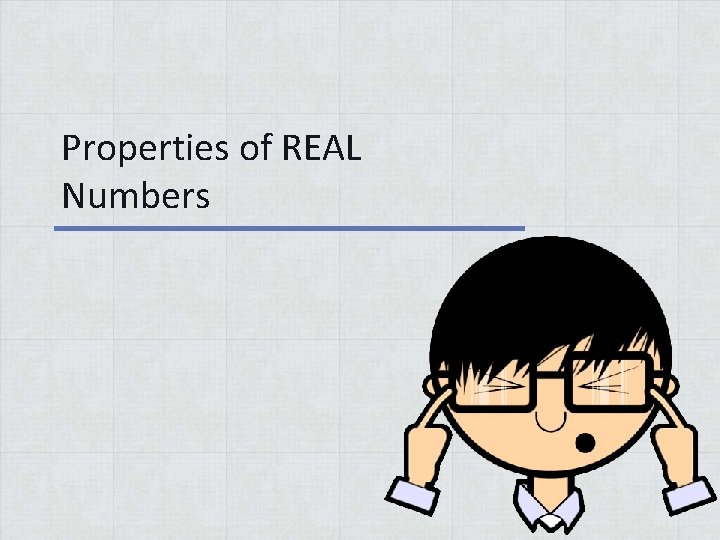Properties of REAL Numbers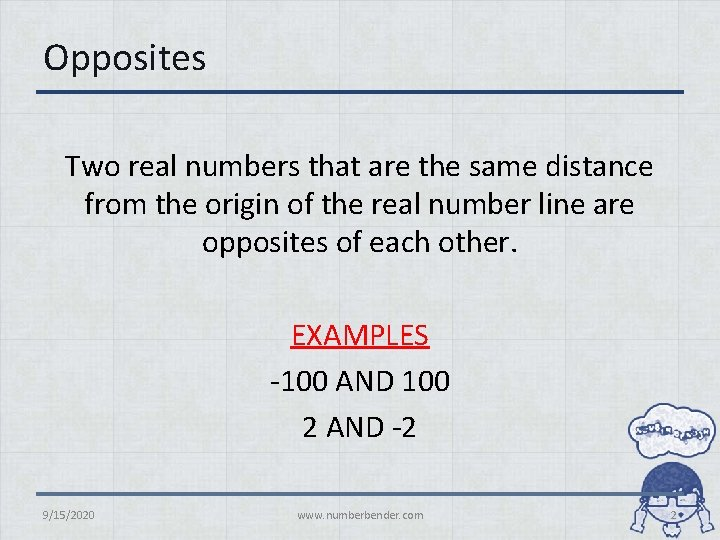Opposites Two real numbers that are the same distance from the origin of the real number line are opposites of each other. EXAMPLES -100 AND 100 2 AND -2 9/15/2020 www. numberbender. com 2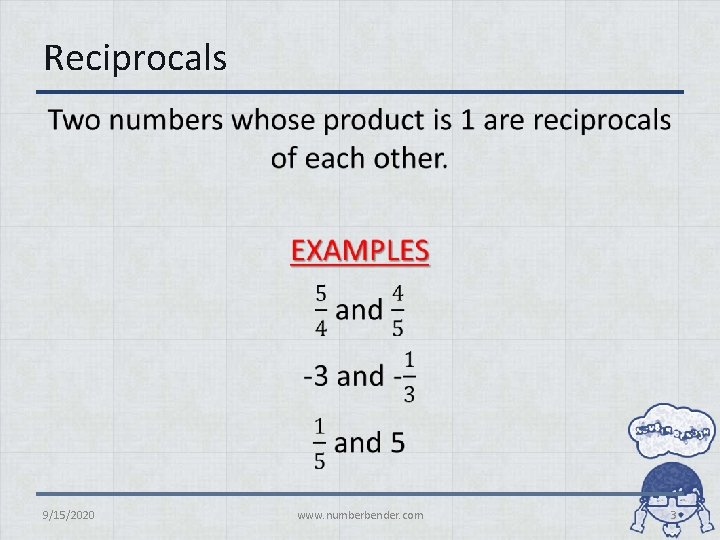Reciprocals • 9/15/2020 www. numberbender. com 3Absolute Value • 9/15/2020 www. numberbender. com 4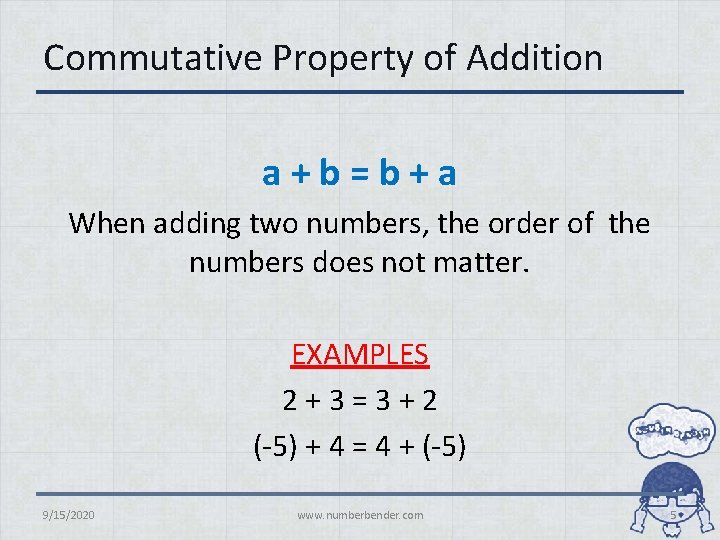Commutative Property of Addition a+b=b+a When adding two numbers, the order of the numbers does not matter. EXAMPLES 2 + 3 = 3 + 2 (-5) + 4 = 4 + (-5) 9/15/2020 www. numberbender. com 5Commutative Property of Multiplication a×b=b×a When multiplying two numbers, the order of the numbers does not matter. EXAMPLES 2 × 3 = 3 × 2 (-3) × 24 = 24 × (-3) 9/15/2020 www. numberbender. com 6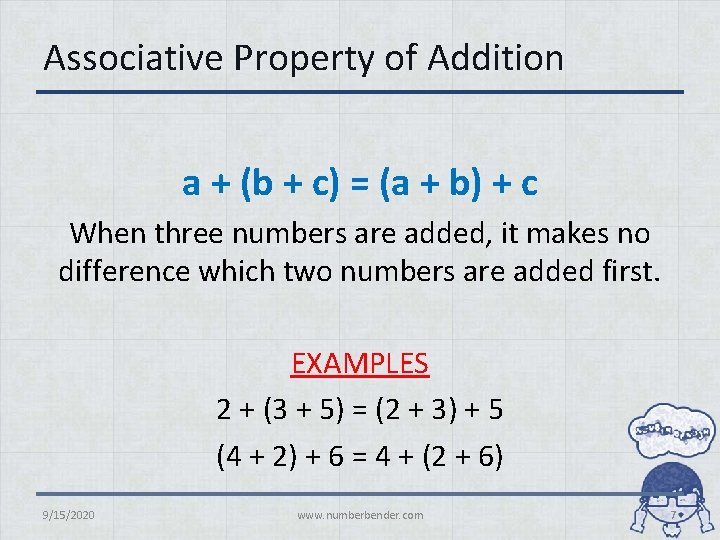Associative Property of Addition a + (b + c) = (a + b) + c When three numbers are added, it makes no difference which two numbers are added first. EXAMPLES 2 + (3 + 5) = (2 + 3) + 5 (4 + 2) + 6 = 4 + (2 + 6) 9/15/2020 www. numberbender. com 7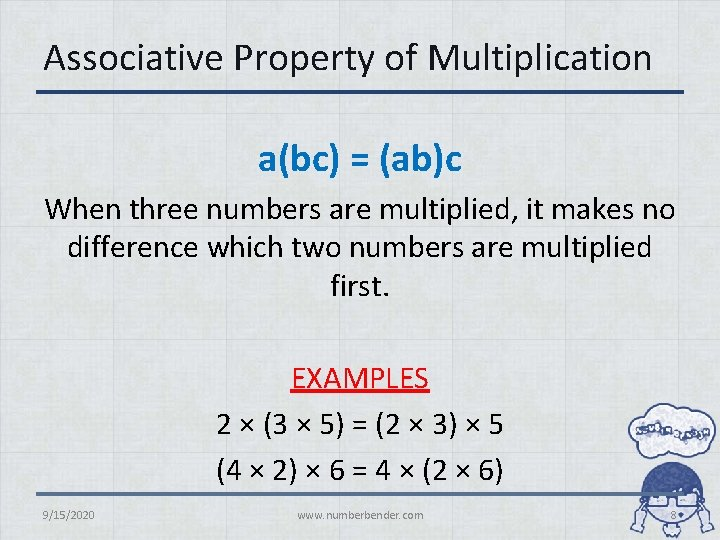Associative Property of Multiplication a(bc) = (ab)c When three numbers are multiplied, it makes no difference which two numbers are multiplied first. EXAMPLES 2 × (3 × 5) = (2 × 3) × 5 (4 × 2) × 6 = 4 × (2 × 6) 9/15/2020 www. numberbender. com 8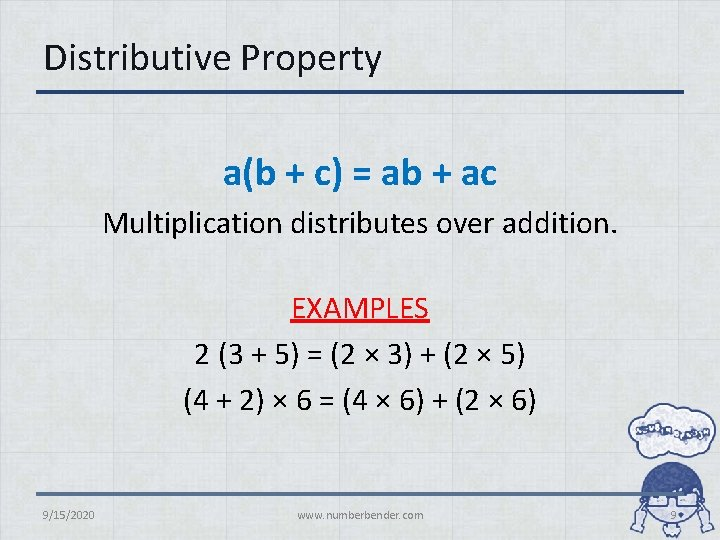Distributive Property a(b + c) = ab + ac Multiplication distributes over addition. EXAMPLES 2 (3 + 5) = (2 × 3) + (2 × 5) (4 + 2) × 6 = (4 × 6) + (2 × 6) 9/15/2020 www. numberbender. com 9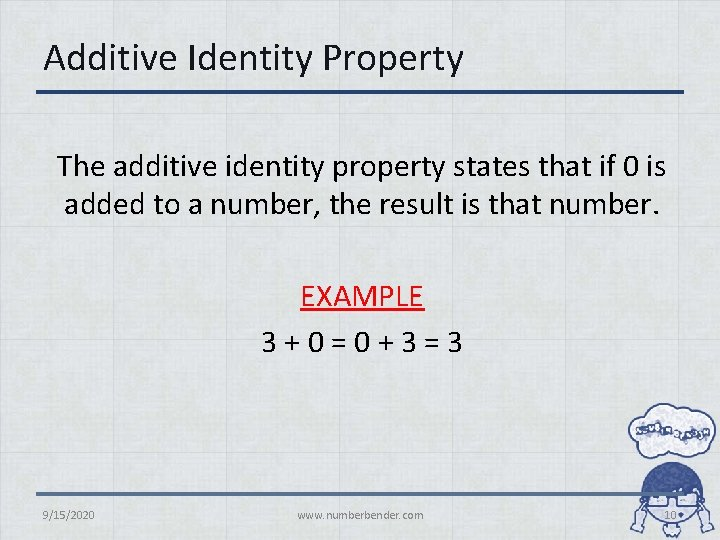Additive Identity Property The additive identity property states that if 0 is added to a number, the result is that number. EXAMPLE 3 + 0 = 0 + 3 = 3 9/15/2020 www. numberbender. com 10Multiplicative Identity Property The multiplicative identity property states that if a number is multiplied by 1, the result is that number. EXAMPLE 5 × 1 = 1 × 5 = 5 9/15/2020 www. numberbender. com 11Additive Inverse Property The additive inverse property states that opposites add to zero. EXAMPLE 7 + (-7) = 0 and -4 + 4 = 0 9/15/2020 www. numberbender. com 12Multiplicative Inverse Property • 9/15/2020 www. numberbender. com 13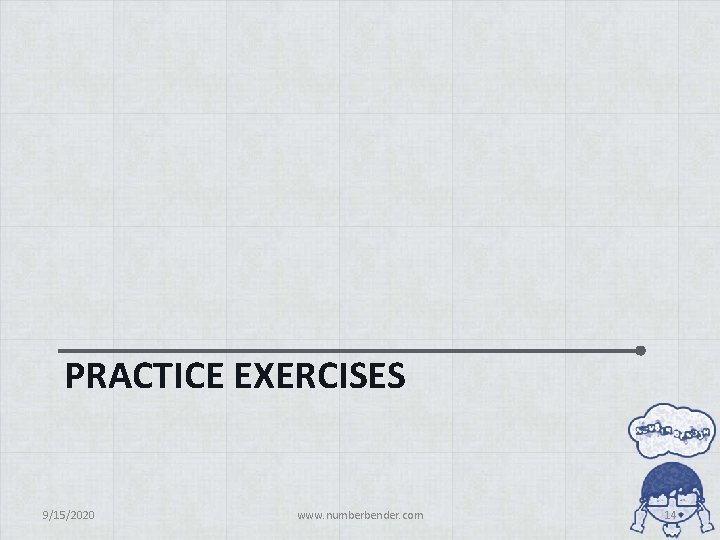PRACTICE EXERCISES 9/15/2020 www. numberbender. com 14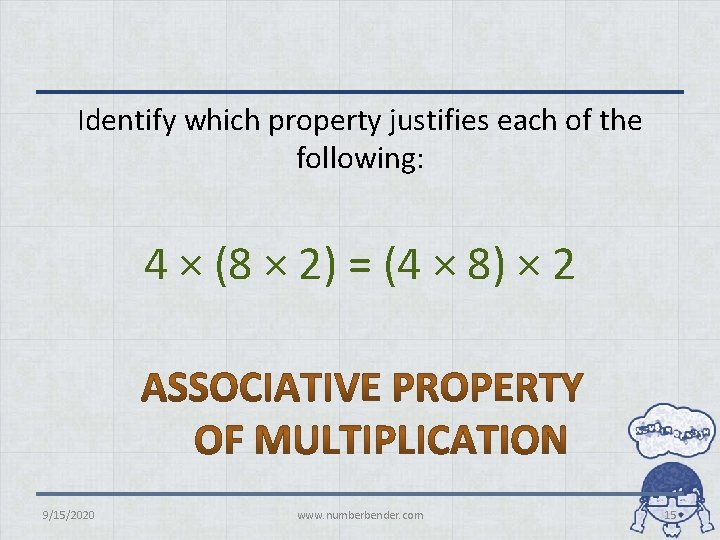Identify which property justifies each of the following: 4 × (8 × 2) = (4 × 8) × 2 9/15/2020 www. numberbender. com 15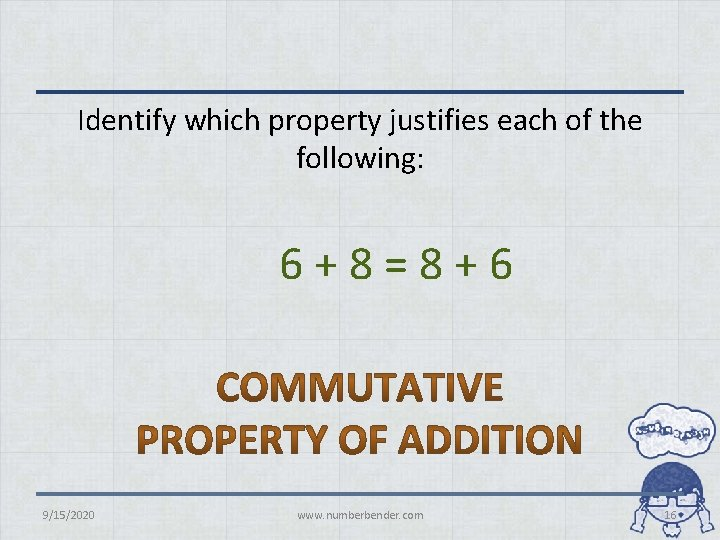Identify which property justifies each of the following: 6 + 8 = 8 + 6 9/15/2020 www. numberbender. com 16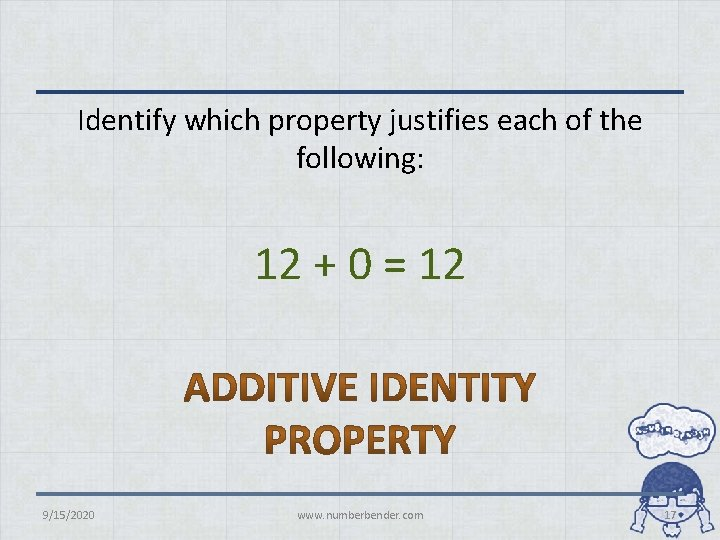Identify which property justifies each of the following: 12 + 0 = 12 9/15/2020 www. numberbender. com 17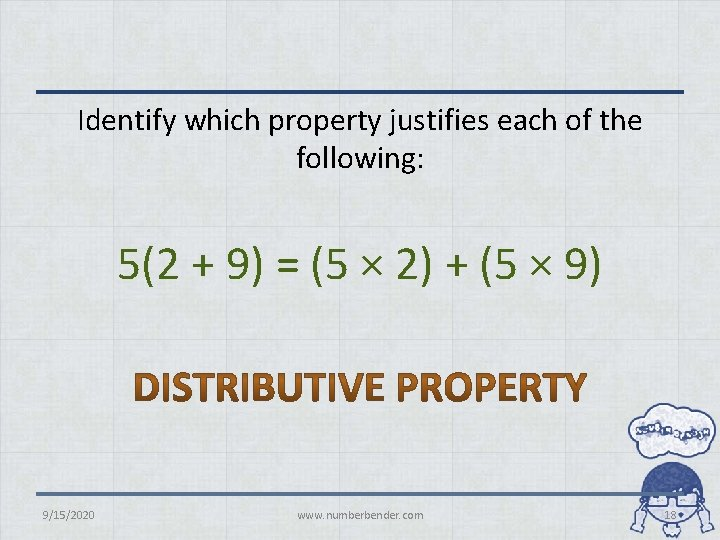Identify which property justifies each of the following: 5(2 + 9) = (5 × 2) + (5 × 9) 9/15/2020 www. numberbender. com 18• 9/15/2020 www. numberbender. com 19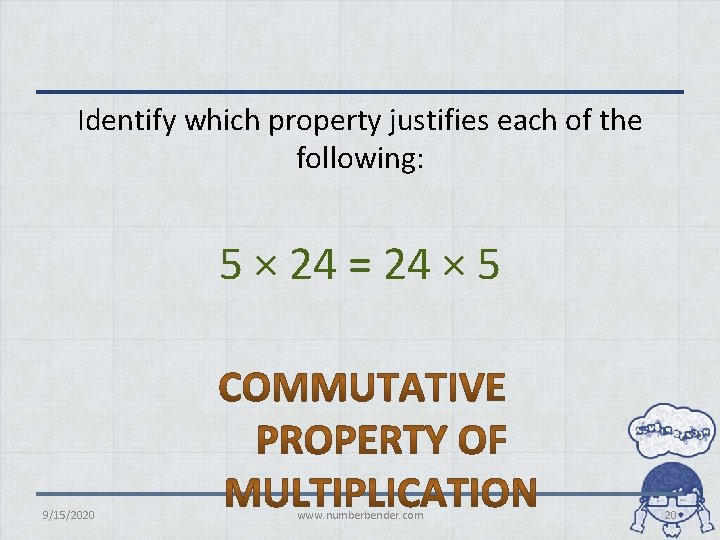Identify which property justifies each of the following: 5 × 24 = 24 × 5 9/15/2020 www. numberbender. com 20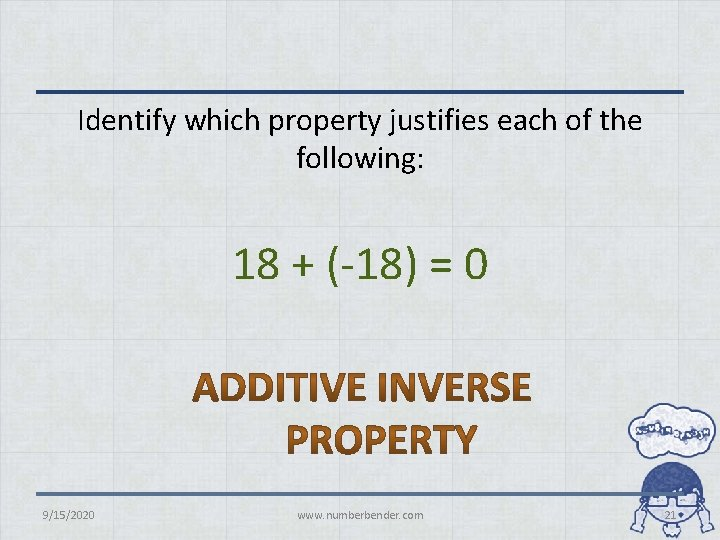Identify which property justifies each of the following: 18 + (-18) = 0 9/15/2020 www. numberbender. com 21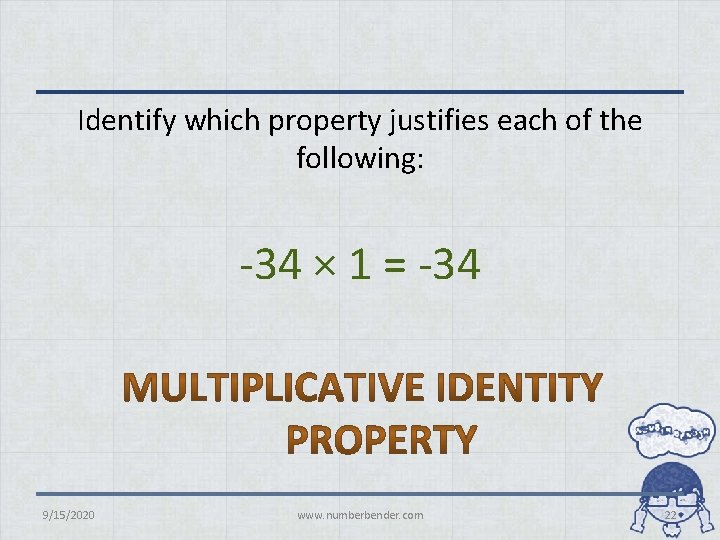Identify which property justifies each of the following: -34 × 1 = -34 9/15/2020 www. numberbender. com 22